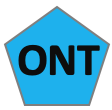# Quality Control using MinION_QCAnother tool for visualisation and QC of nanopore data is MinIONQC. In comparison to PycoQC it is able to compare multiple sequencing runs, e.g., to compare different library preparation or DNA extraction protocols used.

Let’s compare the three summary files in the summaries directory using MinIONQC. From within the qc_practical folder call the MinIONQC.R script:

``````MinIONQC.R –i qc_practical/summaries -o qc_practical/minion_qc
``````

The command above will call MinIONQC which will search in the directory qc_tutorial/summaries for sequencing_summary.txt files.

Output will be written to directory qc_practical/minion_qc:

• One directory for plots and statistics of each of the sequencing runs in directory summaries
• One directory with combined statistics and comparison plots

We’re interested in the results from the combined directory. It contains

• A summary.yaml file. This is a text file with summary statistics for each run and the combined runs
• PNG figures that show plots of all the data together combined (names starting with combined_ )
• PNG figures with plots comparing the different runs

To have a look at the summary.yaml file you can use the command more :

``````more summary.yaml
``````

It will show you combined statistics as well as stats for each of the analysed runs in the summaries directory.

To open the PNG files either double-click the icon in the GUI or use the program eog on the command-line, e.g.:

``````eog combined_length_histogram.png &
``````

Have a look at the different plots, especially the comparison plots, e.g.,

``````eog yield_over_time.png &
``````
1. Does the yield over time increase or decrease? Is any of the runs more consistent than the others?
2. Inspect the plot q_by_hour.png and check the quality of the reads of each run over time. Assuming that the runs represent different library preparation protocols, would you favour any of them? Why?
The above command assumes that you are in directory /home/course_user/course_data/practicals/qc_tutorial/minion_qc/combinedQC. If you want to open the images from within another directory you will have to give the path to the image. For example, if you are in the course_data directory you will have to type
eog qc_tutorial/minion_qc/combinedQC/yield_over_time.png &

The “&” in the commands above will open the image with eog “in the background”. This means that you can continue using the terminal you just typed the command into. Without the “&” the terminal will be blocked until you close eog. Try it if you want.